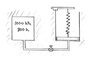# Thermodynamics Closed System Energy Balance

• SweetBabyLou
In summary, the professor was trying to solve a problem where an initial pressure of 200kPa is needed to raise a piston, and when the valve is opened, part of helium flows into the cylinder and pushes the piston up. The final pressure and temperature of the helium is determined by how much helium flowed into the cylinder and how much the piston compressed the spring.

#### SweetBabyLou

Hi everyone,

I am currently reviewing for a thermodynamics exam and have come across a difficult problem while studying. Here it is:

An insulated tank containing helium, a monatomic gas, at P1t = 1000kPa and T1 = 800K is connected to an initially evacuated insulated piston-cylinder device. The mass of the piston is such that an initial pressure in the cylinder of P1c = 200kPa is required to raise the piston at first. Also at the initial state, a linear spring (F~x) is touching the piston but exerts no force on it at first. Now the valve is opened slightly, and part of the helium flows to the cylinder, pushing the piston up and compressing the spring. This process continues until the colume of the helium has doubled. The final state is in mechanical and thermal equilibrium. Assuming helium behaves as an ideal gas, determine:

a) the final pressure of helium P2 (in kPa)

b) the final temperature of the helium T2 (in K)

c) Also, if the initial temperature of T1 had been doubled to 1600K, explain what effect this would have had on the final pressure P2 in part a.

## Homework Statement

The problem gives us a few values to start out with:
P1t = 1000kPa
T1 = 800K
P1c = 200kPa

## Homework Equations

So for this problem, started with the general energy balance equation:
ΔU + ΔKE + ΔPE = Q - W

Since the tank is insulated, I assumed that the process is adiabatic, and thus Q = 0.
I also assumed that ΔKE = ΔPE = 0. With these assumptions, the balance equation becomes:

ΔU = -W → ΔU + W = 0

## The Attempt at a Solution

I tried every which way to try and find a relationship between the values given and specific volumes, specific pressures, enthalpy, etc. I also tried using the hint at the ideal gas law to formulate some sort of useful equation. The only thing I could get from this was that:

since volume doubles, V1 = 1/2 V2.

With P1V1 = mRT1
and P2V2 = mRT2

I took out the m and R term (since they're constant) and got P1/P2 * V1/V2 = T1/T2

isolating the volume term, I get:
V1/V2 = P2/P1 * T1/T2

Since V2 is double V1, V1/V2 = 1/2

∴1/2 = P2/P1 * T1/T2

When I plug in the given information, I can say that:

T2 = 1600K/1000kPa * (P2)

I wanted to try and substitute this value of T2 into one of the equations, but by this point, my brain has been wracked with trying to figure all this out. I am currently at an impasse on where I went wrong with this problem (most likely at the beginning) and looking for some advice on where/how I should rethink this problem. It should be an easy problem, save that no masses of helium have been given (and, of course, helium is not represented data in the back appendices of the book).

I'm sorry to bother all of you with this problem, it may even be a trivial question that's just teasing me under my nose. Any help or advice would be greatly appreciated. Attached below is the professor's diagram of the problem.

Thanks

#### Attachments

•Closed System.JPG
7.9 KB · Views: 648
i worked out the problem, and realized that i needed K(spring const) and cross section area of the piston to solve this problem.
i think everything worked out is correct.
the question says everyhting is in equilibrium at the end which means,
Pf*area=kx+200
the final temp can be found out
using first law Q=U+W

oneomega said:
i worked out the problem, and realized that i needed K(spring const) and cross section area of the piston to solve this problem.
i think everything worked out is correct.
the question says everyhting is in equilibrium at the end which means,
Pf*area=kx+200
the final temp can be found out
using first law Q=U+W

You don't need to know the spring constant, and you don't need to know the cross section area of the piston to solve this problem. Let A be the cross sectional area, and let k be the spring constant (and let m be the mass of the piston). Do a force balance on the piston in the initial state. Its weight is mg and the force acting on it from below is 200A. If the piston rises to a height h, the work done in raising the piston is mgh = 200 Ah. But Ah = V, where V is the volume increase. So the work done to raise the piston is 200 V. Now do a similar kind of analysis for the spring to determine how much work is required to raise the piston to h against against variable spring force. Your result should involve the final pressure Pf, the 200 kPa pressure, and the volume increase V. Now add the two amounts of work to get the total work done by the system.

•1 person